## Welcome to OnlineConversion.com

How do I read a ruler?

Terminology
A ruler used to be called a rule, and rulers would be rules. Today, the more commonly found term is ruler. The dictionary defines both the term rule and ruler, so either can be used, and for this document I will only use the term ruler.

Metric Rulers
Metric rulers are fairly easy to read. They deal with centimeters and millimeters only. You wont have to worry much about fractions.

Take a look at the following Metric Ruler.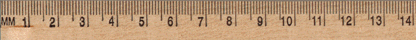The larger lines with numbers are centimeters, and the smallest lines are millimeters. Since millimeters are 1/10th of a centimeter, if you measure 7 marks after a centimeter, it is 1.7 centimeters long.

English Rulers
English rulers, are much more difficult to read. Mostly because they deal with fractions, which are a bit more dificult to learn.

Take a look at the following English Rulers.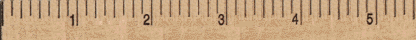A ruler marked in 8ths. Every mark is 1/8th of an inch.A ruler marked in 16ths. Every mark is 1/16th of an inch.

 The center mark between numbers is 1/2.The red lines on these rulers are marked at 1/2, and 1.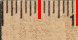The next smallest marks on a ruler are 1/4ths.The red marks on these rulers are at 1/4, 1/2, 3/4, and 1. (1/2 is the same as 2/4)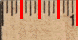The next smallest marks on a ruler are 1/8ths.The red marks on these rulers are at 1/8, 1/4, 3/8, 1/2, 5/8, 3/4, 7/8, and 1.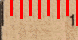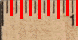The next smallest mark, if there are any, are 1/16ths.The red marks on this ruler are at 1/16, 1/8, 3/16, 1/4, 5/16, 3/8, 7/16, 1/2, 9/16, 5/8, 11/16, 3/4, 13/16, 7/8, 15/16, and 1.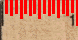When marking down a distance from a ruler, mark the whole inch, followed by a space, then the fraction of an inch.

For example, 1 1/2, or 2 3/8.

When entering fractions into the conversion calculators, you have to separate the whole number and the fraction with a + sign.

For example, 1+1/2, or 2+3/8.

### BookMark Us

It may come in handy.

Are you bored?

Try the Fun Stuff